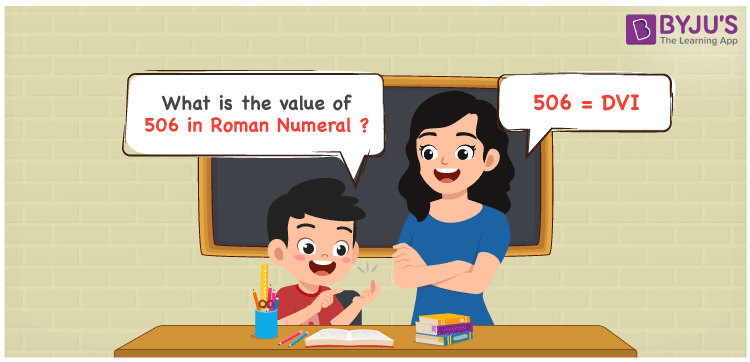DVI is 506 in Roman numerals. To convert 506 to Roman Numerals, first write 506 in expanded form, i.e. 506 = 500 + 5 + 1, then replace the changed numbers with their appropriate roman numerals, resulting in 506 = D + V + I = DVI. In this article, we’ll show you how to convert 506 to Roman numerals accurately.

 Number Roman Numeral 506 DVI## How to Write 506 in Roman Numerals?

In order to write 506 in Roman Numerals, it has to be written in expanded form, i.e.

506 = 500 + 5 + 1

506 = D + V + I

506 = DVI

## Video Lesson on Roman Numerals## Frequently Asked Questions on 506 in Roman Numerals

Q1

### Write 506 in Roman Numerals.

In Roman Numerals, 506 is written as DVI.
Q2

### How to convert 506 into Roman Numerals?

The conversion of 506 into Roman Numerals includes breaking the number according to its place value.
Hundreds = 500 = D
Ones = 6 = VI
Number = 500 + 6 = D + VI = DVI
Q3

### What does 506 in Roman Numerals Mean?

To write 506 in roman numerals, we will first express 506 in expanded form. 506 = 500 + 5 + 1 = D + V + I = DVI. Hence, 506 in roman numbers is expressed as DVI.